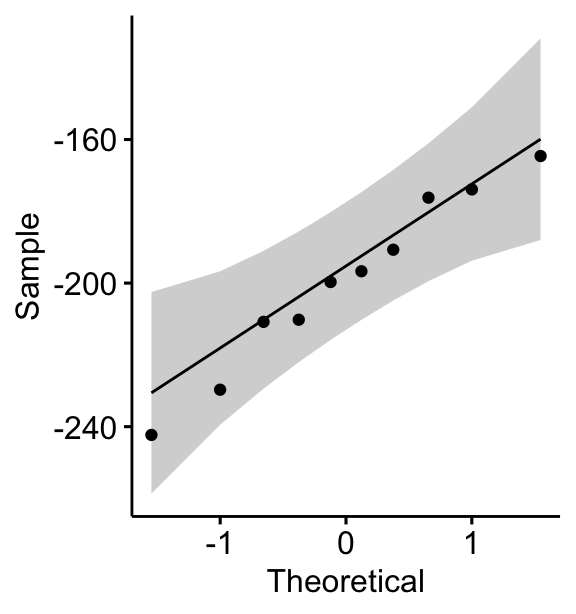# T-Test Essentials: Definition, Formula and Calculation

## Paired T-Test Assumptions

This article describes the paired t-test assumptions and provides examples of R code to check whether the assumptions are met before calculating the t-test. This also referred as:

• paired sample t test assumptions,
• assumptions for matched pairs t test and
• assumptions of dependent t test

The procedure of the paired t-test analysis is as follow:

1. Calculate the difference ($d$) between each pair of value
2. Compute the mean ($m$) and the standard deviation ($s$) of $d$
3. Compare the average difference to 0. If there is any significant difference between the two pairs of samples, then the mean of d ($m$) is expected to be far from 0.

Contents:

#### Related Book

Practical Statistics in R II - Comparing Groups: Numerical Variables

## Assumptions

The paired samples t-test assume the following characteristics about the data:

• the two groups are paired.
• No significant outliers in the difference between the two related groups
• Normality. the difference of pairs follow a normal distribution.

In this section, we’ll perform some preliminary tests to check whether these assumptions are met.

## Check paired t-test assumptions in R

### Prerequisites

Make sure you have installed the following R packages:

• tidyverse for data manipulation and visualization
• ggpubr for creating easily publication ready plots
• rstatix provides pipe-friendly R functions for easy statistical analyses.
• datarium: contains required data sets for this chapter.

library(tidyverse)
library(ggpubr)
library(rstatix)

### Demo data

Here, we’ll use a demo dataset mice2 [datarium package], which contains the weight of 10 mice before and after the treatment.

# Wide format
data("mice2", package = "datarium")
head(mice2, 3)
##   id before after
## 1  1    187   430
## 2  2    194   404
## 3  3    232   406
# Transform into long data:
# gather the before and after values in the same column
mice2.long <- mice2 %>%
gather(key = "group", value = "weight", before, after)
head(mice2.long, 3)
##   id  group weight
## 1  1 before    187
## 2  2 before    194
## 3  3 before    232

First, start by computing the difference between groups:

mice2 <- mice2 %>% mutate(differences = before - after)
head(mice2, 3)
##   id before after differences
## 1  1    187   430        -242
## 2  2    194   404        -210
## 3  3    232   406        -174

### Identify outliers

Outliers can be easily identified using boxplot methods, implemented in the R function identify_outliers() [rstatix package].

mice2 %>% identify_outliers(differences)
##  id          before      after       differences is.outlier  is.extreme
## <0 rows> (or 0-length row.names)

There were no extreme outliers.

Note that, in the situation where you have extreme outliers, this can be due to: 1) data entry errors, measurement errors or unusual values.

You can include the outlier in the analysis anyway if you do not believe the result will be substantially affected. This can be evaluated by comparing the result of the t-test with and without the outlier.

It’s also possible to keep the outliers in the data and perform Wilcoxon test or robust t-test using the WRS2 package.

### Check normality by groups

The normality assumption can be checked by computing the Shapiro-Wilk test for each group. If the data is normally distributed, the p-value should be greater than 0.05.

mice2 %>% shapiro_test(differences) 
## # A tibble: 1 x 3
##   variable    statistic     p
##   <chr>           <dbl> <dbl>
## 1 differences     0.968 0.867

From the output, the two p-values are greater than the significance level 0.05 indicating that the distribution of the data are not significantly different from the normal distribution. In other words, we can assume the normality.

You can also create QQ plots for each group. QQ plot draws the correlation between a given data and the normal distribution.

ggqqplot(mice2, "differences")All the points fall approximately along the (45-degree) reference line, for each group. So we can assume normality of the data.

Note that, if your sample size is greater than 50, the normal QQ plot is preferred because at larger sample sizes the Shapiro-Wilk test becomes very sensitive even to a minor deviation from normality.

In the situation where the data are not normally distributed, it’s recommended to use the non parametric Wilcoxon test.

## Related article

T-test in R